# B.Tech. (SEM-II) THEORY EXAMINATION 2014-15 COMPUTER SYSTEM & PROGRAMMING IN C

Time: 3hrs
Total Marks: 100

Note:-

• Attempt all questions. Marks are indicated against each question.
• Assume suitable data wherever necessary.

### 2. Attempt any TWO parts: (10*2 = 20)

1. What do you understand by ASCII value of a character? Can we use expressions including both integer data type and character data type? Justify your answer.

ASCII is American Standard Code for Information Interchange. ASCII is a character-encoding scheme and it was the first character encoding standard.ASCII uses 7 bits to represent a character. It has 128 code points, 0 through 127. It is a code for representing English characters as numbers, with each letter assigned a number from 0 to 127.

Yes, we can use expressions including both integer data type and character data type. In such expressions, type conversions occur and the character datatype gets converted into its integer value. This integer value is the ASCII value of the character.

Example:

 `// An example to show we can use ` `// expressions including both  ` `// integer data type and character data type ` ` `  `#include ` ` `  `int` `main() ` `{ ` `    ``int` `x = 10; ``// integer x ` `    ``char` `y = ``'a'``; ``// character c ` `    ``int` `z; ` ` `  `    ``// y implicitly converted to int. ASCII ` `    ``// value of 'a' is 97 ` `    ``z = x + y; ` ` `  `    ``printf``(``"z = %d"``, z); ` `    ``return` `0; ` `} `

Output:

`z = 107`
2. Write the difference between type conversion and type casting. What are the escape sequences characters?

In C programming language, there are 256 numbers of characters in character set. The entire character set is divided into 2 parts i.e. the ASCII characters set and the extended ASCII characters set. But apart from that, some other characters are also there which are not the part of any characters set, known as ESCAPE characters.

List of Escape Sequences

```\a    Alarm or Beep
\b    Backspace
\f    Form Feed
\n    New Line
\r    Carriage Return
\t    Tab (Horizontal)
\v    Vertical Tab
\\    Backslash
'    Single Quote
\"    Double Quote
\?    Question Mark
\ooo  octal number
\0    Null```

Example:

 `// C program to illustrate ` `// \b escape sequence ` `#include ` `int` `main(``void``) ` `{ ` `    ``// \b - backspace character transfers ` `    ``// the cursor one character back with ` `    ``// or without deleting on different ` `    ``// compilers. ` `    ``printf``(``"Hello Geeks\b\b\b\bF"``); ` `    ``return` `(0); ` `} `

Output:

```The output is dependent upon compiler.
```
3. Convert the following number into:

(i) (11010.0110)2 = ()10

(11010.0110)2
= (26.375)10

(ii) (110101011.0110110)2 = ()8

(110101011.0110110)2
= (653.33)8

(iii) (2B6D)16 = ()2

(2B6D)16
= (10101101101101)2

(iv) (AB4F.C1)16 = ()10

(AB4F.C1)16
= (43855.75390625)10

(v) (54)6 = ()4

(54)6
= (202)4

### 3. Attempt any TWO parts: (10*2 = 20)

1. Give the loop statement to print the following sequence of integers:
-6 -4 -2 0 2 4 6

 `#include ` ` `  `int` `main() ` `{ ` ` `  `    ``int` `i = 0; ` ` `  `    ``for` `(i = -6; i <= 6; i += 2) ` `        ``printf``(``"%d "``, i); ` ` `  `    ``return` `0; ` `} `

Output:

`-6 -4 -2 0 2 4 6 `
2. What are the main principles of recursion?

The process in which a function calls itself directly or indirectly is called recursion and the corresponding function is called as recursive function. Using recursive algorithm, certain problems can be solved quite easily. Examples of such problems are Towers of Hanoi (TOH), Inorder/Preorder/Postorder Tree Traversals, DFS of Graph, etc.

What is base condition in recursion?
In recursive program, the solution to base case is provided and solution of bigger problem is expressed in terms of smaller problems.

```int fact(int n)
{
if (n < = 1) // base case
return 1;
else
return n*fact(n-1);
}
```

In the above example, base case for n < = 1 is defined and larger value of number can be solved by converting to smaller one till base case is reached.

3. If int a=2, b=3, x=0; Find the value of x=(++a, b+=a)

Output: x = 6
Explanation:

• As a = 2, b = 3 and x = 0 intitially.
• Therefore,
x = (++a, b+=a)
= ((++a), b+=a)
= (b+=a)…..Now a = 3
= (b = b+a)
= (b = 3+3)
= (b = 6)
= (6)
x = 6

### 4. Attempt any TWO parts: (10*2 = 20)

1. What are the different types of operators in C language and also write down the difference between the associativity and precedence of operators.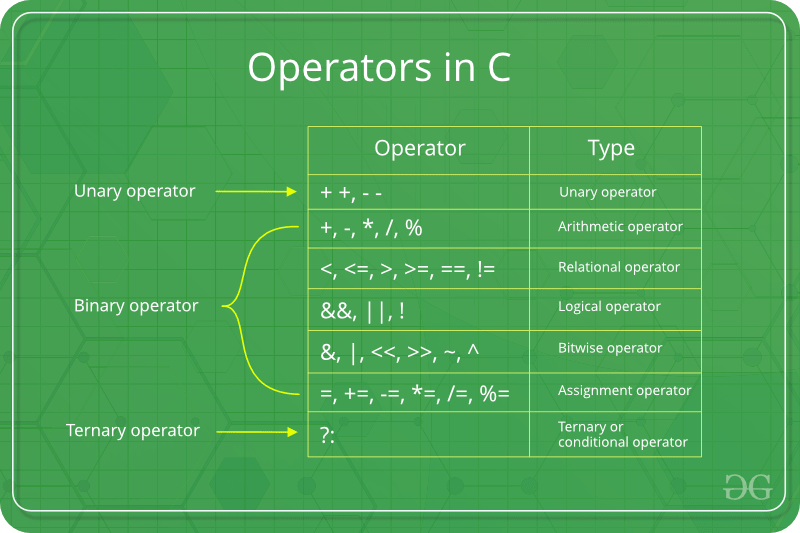Operators are the foundation of any programming language. Thus the functionality of C/C++ programming language is incomplete without the use of operators. We can define operators as symbols that helps us to perform specific mathematical and logical computations on operands. In other words we can say that an operator operates the operands.
For example, consider the below statement:

```c = a + b;
```

Here, ‘+’ is the operator known as addition operator and ‘a’ and ‘b’ are operands. The addition operator tells the compiler to add both of the operands ‘a’ and ‘b’. C/C++ has many built-in operator types and they can be classified as:

• Arithmetic Operators: These are the operators used to perform arithmetic/mathematical operations on operands. Examples: (+, -, *, /, %, ++, –).
• Relational Operators: Relational operators are used for comparison of the values of two operands. For example: checking if one operand is equal to the other operand or not, an operand is greater than the other operand or not etc. Some of the relational operators are (==, >=, <= ). To learn about each of these operators in details go to this link.
• Logical Operators:  Logical Operators are used to combine two or more conditions/constraints or to complement the evaluation of the original condition in consideration. The result of the operation of a logical operator is a boolean value either true or false. To learn about different logical operators in details please visit this link.
• Bitwise Operators: The Bitwise operators is used to perform bit-level operations on the operands. The operators are first converted to bit-level and then calculation is performed on the operands. The mathematical operations such as addition, subtraction, multiplication etc. can be performed at bit-level for faster processing. To learn bitwise operators in details, visit this link.
• Assignment Operators: Assignment operators are used to assign value to a variable. The left side operand of the assignment operator is a variable and right side operand of the assignment operator is a value. The value on the right side must be of the same data-type of variable on the left side otherwise the compiler will raise an error.
• Other Operators: Apart from the above operators there are some other operators available in C or C++ used to perform some specific task. Some of them are discussed here:
• sizeof operator: sizeof is a much used in the C/C++ programming language. It is a compile time unary operator which can be used to compute the size of its operand. The result of sizeof is of unsigned integral type which is usually denoted by size_t. Basically, sizeof operator is used to compute the size of the variable. To learn about sizeof operator in details you may visit this link.
• Comma Operator: The comma operator (represented by the token, ) is a binary operator that evaluates its first operand and discards the result, it then evaluates the second operand and returns this value (and type). The comma operator has the lowest precedence of any C operator. Comma acts as both operator and separator. To learn about comma in details visit this link.
• Conditional Operator: Conditional operator is of the form Expression1 ? Expression2 : Expression3 . Here, Expression1 is the condition to be evaluated. If the condition(Expression1) is True then we will execute and return the result of Expression2 otherwise if the condition(Expression1) is false then we will execute and return the result of Expression3. We may replace the use of if..else statements by conditional operators. To learn about conditional operators in details, visit this link.
• (i) What is the role of SWITCH statement in C programming language? Explain with example.

Switch case statements are a substitute for long if statements that compare a variable to several integral values

• The switch statement is a multiway branch statement. It provides an easy way to dispatch execution to different parts of code based on the value of the expression.
• Switch is a control statement that allows a value to change control of execution.

Syntax:

```switch (n)
{
case 1: // code to be executed if n = 1;
break;
case 2: // code to be executed if n = 2;
break;
default: // code to be executed if n doesn't match any cases
}```

Flowchart:Example:

 `// Following is a simple program to demonstrate ` `// syntax of switch. ` `#include ` `int` `main() ` `{ ` `    ``int` `x = 2; ` `    ``switch` `(x) { ` `    ``case` `1: ` `        ``printf``(``"Choice is 1"``); ` `        ``break``; ` `    ``case` `2: ` `        ``printf``(``"Choice is 2"``); ` `        ``break``; ` `    ``case` `3: ` `        ``printf``(``"Choice is 3"``); ` `        ``break``; ` `    ``default``: ` `        ``printf``(``"Choice other than 1, 2 and 3"``); ` `        ``break``; ` `    ``} ` `    ``return` `0; ` `} `

Output:

```Choice is 2
```

Output:

`Choice is 2`
• (ii) Distinguish between:
• 1. actual & formal arguments

• Formal Parameter : A variable and its type as they appear in the prototype of the function or method.
• Actual Parameter : The variable or expression corresponding to a formal parameter that appears in the function or method call in the calling environment.
• 2. global & extern variables

• extern: Extern storage class simply tells us that the variable is defined elsewhere and not within the same block where it is used. Basically, the value is assigned to it in a different block and this can be overwritten/changed in a different block as well. So an extern variable is nothing but a global variable initialized with a legal value where it is declared in order to be used elsewhere. It can be accessed within any function/block. Also, a normal global variable can be made extern as well by placing the ‘extern’ keyword before its declaration/definition in any function/block. This basically signifies that we are not initializing a new variable but instead we are using/accessing the global variable only. The main purpose of using extern variables is that they can be accessed between two different files which are part of a large program. For more information on how extern variables work, have a look at this link.
• Global Variables: As the name suggests, Global Variables can be accessed from any part of the program.
• They are available through out the life time of a program.
• They are declared at the top of the program outside all of the functions or blocks.
• Declaring global variables: Global variables are usually declared outside of all of the functions and blocks, at the top of the program. They can be accessed from any portion of the program.
2. Describe call by value and call by reference with example.

There are different ways in which parameter data can be passed into and out of methods and functions. Let us assume that a function B() is called from another function A(). In this case A is called the “caller function” and B is called the “called function or callee function”. Also, the arguments which A sends to B are called actual arguments and the parameters of B are called formal arguments.

Important methods of Parameter Passing

1. Pass By Value : This method uses in-mode semantics. Changes made to formal parameter do not get transmitted back to the caller. Any modifications to the formal parameter variable inside the called function or method affect only the separate storage location and will not be reflected in the actual parameter in the calling environment. This method is also called as call by value.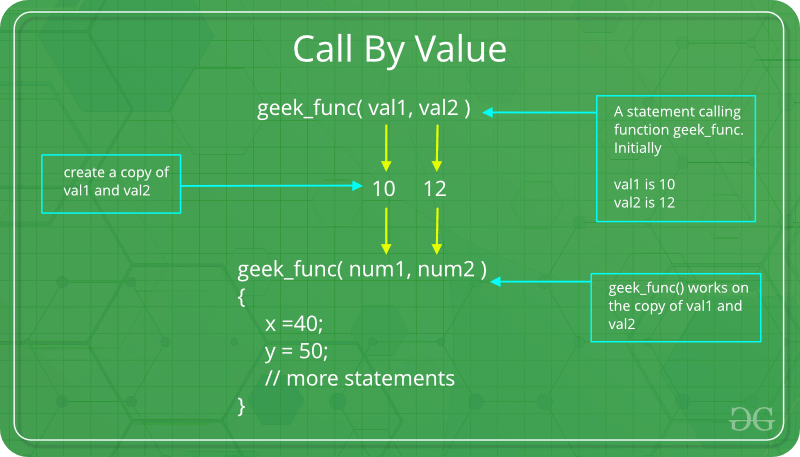`// C program to illustrate ` `// call by value ` `#include ` ` `  `void` `func(``int` `a, ``int` `b) ` `{ ` `    ``a += b; ` `    ``printf``(``"In func, a = %d b = %d\n"``, a, b); ` `} ` `int` `main(``void``) ` `{ ` `    ``int` `x = 5, y = 7; ` ` `  `    ``// Passing parameters ` `    ``func(x, y); ` `    ``printf``(``"In main, x = %d y = %d\n"``, x, y); ` `    ``return` `0; ` `} `

Output:

```In func, a = 12 b = 7
In main, x = 5 y = 7
```

Languages like C, C++, Java support this type of parameter passing. Java in fact is strictly call by value.
Shortcomings:

• Inefficiency in storage allocation
• For objects and arrays, the copy semantics are costly
2. Pass by reference(aliasing) : This technique uses in/out-mode semantics. Changes made to formal parameter do get transmitted back to the caller through parameter passing. Any changes to the formal parameter are reflected in the actual parameter in the calling environment as formal parameter receives a reference (or pointer) to the actual data. This method is also called as <em>call by reference. This method is efficient in both time and space.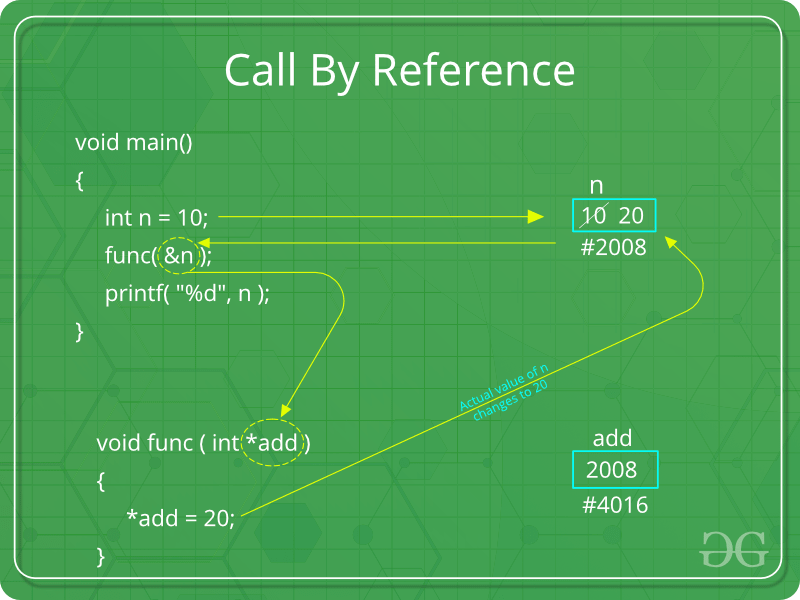`// C program to illustrate ` `// call by reference ` `#include ` ` `  `void` `swapnum(``int``* i, ``int``* j) ` `{ ` `    ``int` `temp = *i; ` `    ``*i = *j; ` `    ``*j = temp; ` `} ` ` `  `int` `main(``void``) ` `{ ` `    ``int` `a = 10, b = 20; ` ` `  `    ``// passing parameters ` `    ``swapnum(&a, &b); ` ` `  `    ``printf``(``"a is %d and b is %d\n"``, a, b); ` `    ``return` `0; ` `} `

Output:

```a is 20 and b is 10
```

### 5. Attempt any TWO parts: (10*2 = 20)

1. Write a program in C language to generate the Fibonacci series.

Program in C to generate the Fibonacci series:

 `// Fibonacci Series using Recursion ` ` `  `#include ` ` `  `int` `fib(``int` `n) ` `{ ` `    ``if` `(n <= 1) ` `        ``return` `n; ` `    ``return` `fib(n - 1) + fib(n - 2); ` `} ` ` `  `int` `main() ` `{ ` `    ``int` `n = 9; ` `    ``printf``(``"%d"``, fib(n)); ` `    ``getchar``(); ` `    ``return` `0; ` `} `

Output:

```34
```
2. What do you mean by dynamic memory allocation? Explain the following functions in detail: free and calloc

Dynamic Memory Allocation in C: It can be defined as a procedure in which the size of a data structure (like Array) is changed during the runtime.

C provides some functions to achieve these tasks. There are 4 library functions provided by C defined under <stdlib.h> header file to facilitate dynamic memory allocation in C programming. They are:

1. malloc()
2. calloc()
3. free()
4. realloc()

Lets see each of them in detail.

1. calloc()

“calloc” or “contiguous allocation” method is used to dynamically allocate the specified number of blocks of memory of the specified type. It initializes each block with a default value ‘0’.

Syntax:

```ptr = (cast-type*)calloc(n, element-size);

For Example:
ptr = (float*) calloc(25, sizeof(float));

This statement allocates contiguous space in memory
for 25 elements each with the size of float.
```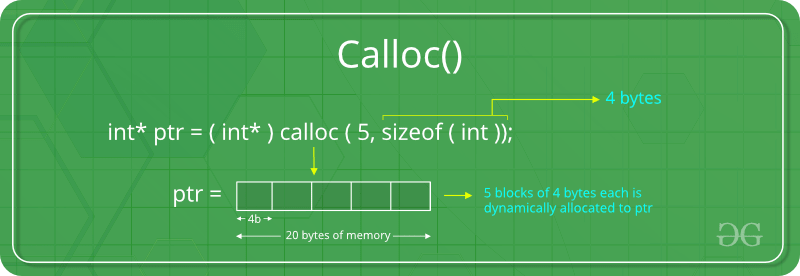If the space is insufficient, allocation fails and returns a NULL pointer.

Example:

 `#include ` `#include ` ` `  `int` `main() ` `{ ` ` `  `    ``// This pointer will hold the ` `    ``// base address of the block created ` `    ``int``* ptr; ` `    ``int` `n, i, sum = 0; ` ` `  `    ``// Get the number of elements for the array ` `    ``n = 5; ` `    ``printf``(``"Enter number of elements: %d\n"``, n); ` ` `  `    ``// Dynamically allocate memory using calloc() ` `    ``ptr = (``int``*)``calloc``(n, ``sizeof``(``int``)); ` ` `  `    ``// Check if the memory has been successfully ` `    ``// allocated by malloc or not ` `    ``if` `(ptr == NULL) { ` `        ``printf``(``"Memory not allocated.\n"``); ` `        ``exit``(0); ` `    ``} ` `    ``else` `{ ` ` `  `        ``// Memory has been successfully allocated ` `        ``printf``(``"Memory successfully allocated using calloc.\n"``); ` ` `  `        ``// Get the elements of the array ` `        ``for` `(i = 0; i < n; ++i) { ` `            ``ptr[i] = i + 1; ` `        ``} ` ` `  `        ``// Print the elements of the array ` `        ``printf``(``"The elements of the array are: "``); ` `        ``for` `(i = 0; i < n; ++i) { ` `            ``printf``(``"%d, "``, ptr[i]); ` `        ``} ` `    ``} ` ` `  `    ``return` `0; ` `} `

Output:

```Enter number of elements: 5
Memory successfully allocated using calloc.
The elements of the array are: 1, 2, 3, 4, 5,
```
2. free()

“free” method is used to dynamically de-allocate the memory. The memory allocated using functions malloc() and calloc() are not de-allocated on their own. Hence the free() method is used, whenever the dynamic memory allocation takes place. It helps to reduce wastage of memory by freeing it.

Syntax:

```free(ptr);
```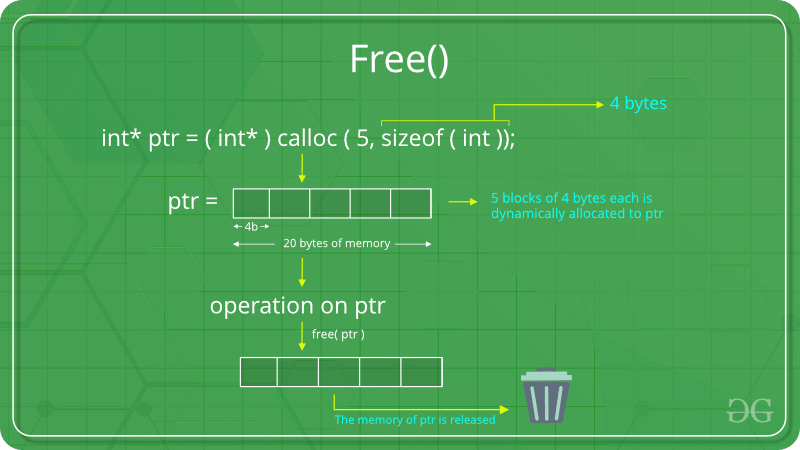Example:

 `#include ` `#include ` ` `  `int` `main() ` `{ ` ` `  `    ``// This pointer will hold the ` `    ``// base address of the block created ` `    ``int` `*ptr, *ptr1; ` `    ``int` `n, i, sum = 0; ` ` `  `    ``// Get the number of elements for the array ` `    ``n = 5; ` `    ``printf``(``"Enter number of elements: %d\n"``, n); ` ` `  `    ``// Dynamically allocate memory using malloc() ` `    ``ptr = (``int``*)``malloc``(n * ``sizeof``(``int``)); ` ` `  `    ``// Dynamically allocate memory using calloc() ` `    ``ptr1 = (``int``*)``calloc``(n, ``sizeof``(``int``)); ` ` `  `    ``// Check if the memory has been successfully ` `    ``// allocated by malloc or not ` `    ``if` `(ptr == NULL || ptr1 == NULL) { ` `        ``printf``(``"Memory not allocated.\n"``); ` `        ``exit``(0); ` `    ``} ` `    ``else` `{ ` ` `  `        ``// Memory has been successfully allocated ` `        ``printf``(``"Memory successfully allocated using malloc.\n"``); ` ` `  `        ``// Free the memory ` `        ``free``(ptr); ` `        ``printf``(``"Malloc Memory successfully freed.\n"``); ` ` `  `        ``// Memory has been successfully allocated ` `        ``printf``(``"\nMemory successfully allocated using calloc.\n"``); ` ` `  `        ``// Free the memory ` `        ``free``(ptr1); ` `        ``printf``(``"Calloc Memory successfully freed.\n"``); ` `    ``} ` ` `  `    ``return` `0; ` `} `

Output:

```Enter number of elements: 5
Memory successfully allocated using malloc.
Malloc Memory successfully freed.

Memory successfully allocated using calloc.
Calloc Memory successfully freed.
```
3. Write a program to add two matrices of dimension 3*3 and store the result in another matrix

 `#include ` `#define N 3 ` ` `  `// This function adds A[][] and B[][], and stores ` `// the result in C[][] ` `void` `add(``int` `A[][N], ``int` `B[][N], ``int` `C[][N]) ` `{ ` `    ``int` `i, j; ` `    ``for` `(i = 0; i < N; i++) ` `        ``for` `(j = 0; j < N; j++) ` `            ``C[i][j] = A[i][j] + B[i][j]; ` `} ` ` `  `int` `main() ` `{ ` `    ``int` `A[N][N] = { { 1, 1, 1 }, ` `                    ``{ 2, 2, 2 }, ` `                    ``{ 3, 3, 3 } }; ` ` `  `    ``int` `B[N][N] = { { 1, 1, 1 }, ` `                    ``{ 2, 2, 2 }, ` `                    ``{ 3, 3, 3 } }; ` ` `  `    ``int` `C[N][N]; ``// To store result ` `    ``int` `i, j; ` `    ``add(A, B, C); ` ` `  `    ``printf``(``"Result matrix is \n"``); ` `    ``for` `(i = 0; i < N; i++) { ` `        ``for` `(j = 0; j < N; j++) ` `            ``printf``(``"%d "``, C[i][j]); ` `        ``printf``(``"\n"``); ` `    ``} ` ` `  `    ``return` `0; ` `} `

Output:

```Result matrix is
2 2 2
4 4 4
6 6 6
```

My Personal Notes arrow_drop_upCheck out this Author's contributed articles.

If you like GeeksforGeeks and would like to contribute, you can also write an article using contribute.geeksforgeeks.org or mail your article to contribute@geeksforgeeks.org. See your article appearing on the GeeksforGeeks main page and help other Geeks.

Please Improve this article if you find anything incorrect by clicking on the "Improve Article" button below.

Article Tags :

Be the First to upvote.

Please write to us at contribute@geeksforgeeks.org to report any issue with the above content.